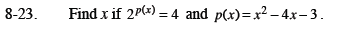Home > CCA2 > Chapter 8 > Lesson 8.1.1 > Problem8-23

8-23.

Find x if 2p(x) = 4 and p(x) = x2 − 4x − 3. Homework Help ✎Since 4 = 2², what does p(x) have to equal?

x = −1, x = 5